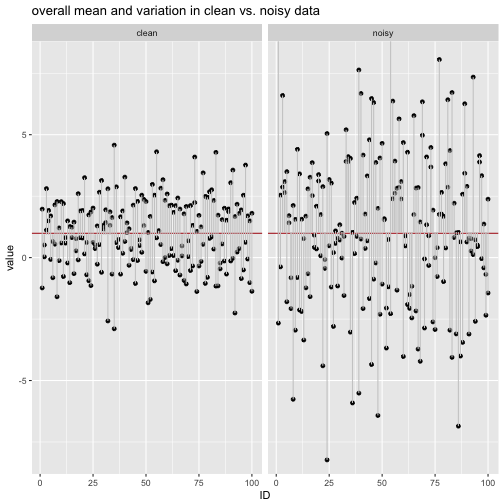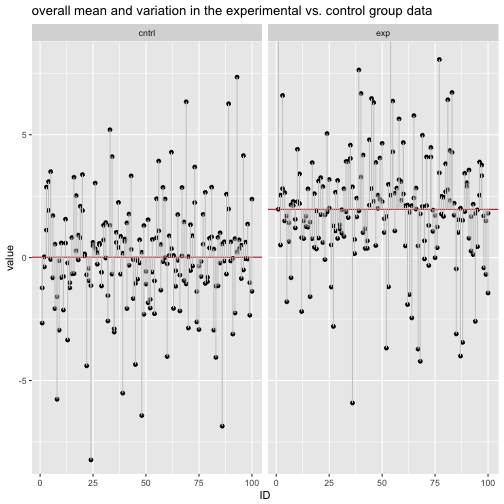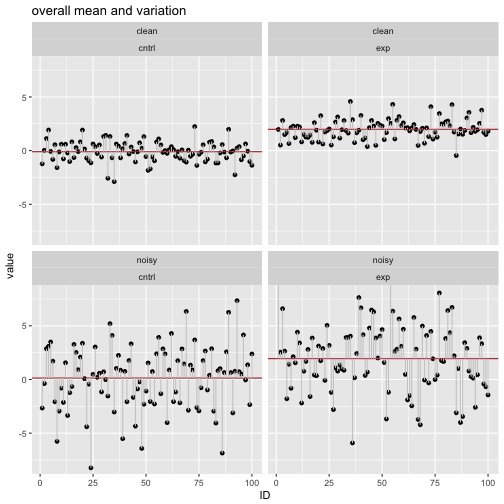# Variance explained vs. variance blurred

Frequently, someones says that some indicator variable X “explains” some proportion of some target variable, Y. What does this actually mean? By “mean” I am trying to find some intuition that “clicks” rather than citing the (well-known) formualas.

library(tidyverse)

n_rows <- 100
set.seed(271828)

df <- data_frame(
exp_clean = rnorm(n = n_rows, mean = 2, sd = 1),
cntrl_clean = rnorm(n = n_rows, mean = 0, sd = 1),
exp_noisy = exp_clean + rnorm(n = n_rows, mean = 0, sd = 3),
cntrl_noisy = cntrl_clean + rnorm(n = n_rows, mean = 0, sd = 3),
ID = 1:n_rows)


Here, we drew 100 cases from the population of the “experimental group” (mue = 2) and 100 cases from the control group (mue = 0). We will investigate the effect of noise on our data. So for both groups we make up noisy data: We just add some random noise on the existing data.

To easily plot the data, it is useful to “gather” or “melt” the data from wide to long format.

df_long <-
df %>%
gather(key = group, value = value, -ID) %>%
separate(col = group, into = c("group", "noise_level"), sep = "_")


# Summary data

It is useful to put some summary data in its own dataframe in a structured way:

df_sum <-
df_long %>%
group_by(group, noise_level) %>%
summarise(value_mean = mean(value)) %>%
ungroup

knitr::kable(df_sum)

group noise_level value_mean
cntrl clean -0.1035629
cntrl noisy 0.1352067
exp clean 1.9834936
exp noisy 1.9421094

# Clean data vs. noisy data

For plotting purposes, it will be handy to have the group means in the main dataframe:

df_sum_noise_level <-
df_sum %>%
select(-group) %>%
group_by(noise_level) %>%
summarise_all(funs(mean))

knitr::kable(df_sum_noise_level)

noise_level value_mean
clean 0.9399653
noisy 1.0386580
df_long_noise_level <- df_long %>%
left_join(df_sum_noise_level, by = "noise_level")


Joining operations basically merge two dataframes matched by some matchings columns (by argument).

Now, let’s plot the data including the mean value and the within variation.

df_long_noise_level %>%
ggplot +
aes(x = ID, y = value) +
facet_wrap(~noise_level) +
geom_point() +
geom_hline(data = df_sum_noise_level, aes(yintercept = mean(value_mean)), color = "firebrick") +
geom_linerange(aes(ymin = value_mean, ymax = value), color = "grey80") +
labs(title = "overall mean and variation in clean vs. noisy data") +
coord_cartesian(ylim = c(-8,8))We see that the the variation (the grey deviation bars) are larger in the noisy data compared to the clean data.

Now let’s compute the average length (MAD) and the variance of the grey bars.

df_long_noise_level %>%
group_by(noise_level) %>%
summarise(value_mad = mean(abs(value - mean(.$value))), value_var = mean(((value - mean(.$value))^2)))

## # A tibble: 2 × 3
##         <chr>     <dbl>     <dbl>
## 1       clean  1.163611  1.963961
## 2       noisy  2.473277  9.985220


# Explaining variable

Now let’s investigate the influence of some “explaining” variable. Say, our data stems from two populations, the experimental condition and the control condtion, with differing means. Assume that the experimental condition has a mean 2 sd higher than in the control condition. Hence, the grouping variable will help to reduce the variation.

First, we compute the summary statistics and join them to the main dataframe.

df_sum_group <-
df_sum %>%
select(-noise_level) %>%
group_by(group) %>%
summarise_all(funs(mean))

knitr::kable(df_sum_group)

group value_mean
cntrl 0.0158219
exp 1.9628015
df_long_group <- df_long %>%
left_join(df_sum_group, by = "group")


Now, plot the data conditioned on the explaining variable, ie., the condition (experimental vs. control).

df_long_group %>%
ggplot +
aes(x = ID, y = value) +
facet_wrap(~group) +
geom_point() +
geom_hline(data = df_sum_group, aes(yintercept = value_mean), color = "firebrick") +
geom_linerange(aes(ymin = value_mean, ymax = value), color = "grey80") +
labs(title = "overall mean and variation in the experimental vs. control group data") +
coord_cartesian(ylim = c(-8,8))Practically speaking, if we measure the deviation to the respective group mean, we see that the deviation (aka, variation within each group) has decreased when considering the explaining variable.

In number parlance:

df_long_group %>%
group_by(group) %>%
summarise(value_mad = mean(abs(value - mean(.$value))), value_var = mean(((value - mean(.$value))^2)))

## # A tibble: 2 × 3
##   <chr>     <dbl>     <dbl>
## 1 cntrl  1.777131  5.626771
## 2   exp  1.859757  6.322410


# Whole picture

Now let’s compare all the four subgroups:

• clean vs. noisy data and
• data from experimental group vs. control group.

Again, we start by adding the group summaries to the main data frame for easier plotting.

df_long_all <- df_long %>%
left_join(df_sum, by = c("group", "noise_level"))


And now we plot the 4 subgroups.

df_long_all %>%
ggplot +
aes(x = ID, y = value) +
facet_wrap(noise_level~group) +
geom_point() +
geom_hline(data = df_sum, aes(yintercept = value_mean), color = "firebrick") +
geom_linerange(aes(ymin = value_mean, ymax = value), color = "grey80") +
labs(title = "overall mean and variation ") +
coord_cartesian(ylim = c(-8,8))Similarly to the previous steps, we calculate the summary statistics for each of the 4 groups.

df_long_all %>%
group_by(noise_level, group) %>%
summarise(value_mad = mean(abs(value - mean(.$value))), value_var = mean(((value - mean(.$value))^2)))

## Source: local data frame [4 x 4]
## Groups: noise_level [?]
##